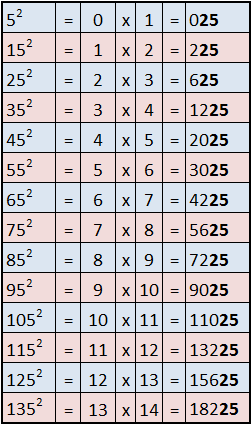Squaring numbers ending in 5

Imagine being asked to find the square (multiply a number by itself) of a number ending in 5. eg (35×35,95×95)

This little trick will help you find the answer in fewer than 3 seconds.

Let us use 65 for example

1.Take the digit(s) preceding 5  and multiply it by the next highest number.

65

6x(6+1)

6×7=42

So the answer will be  42xx

2. Put 25 at the end of the number you have (the 2 xx at the right) . It twill always end in 25

Illustrations

View the bellow examples including how this method can be used for 3 digit numbers as well